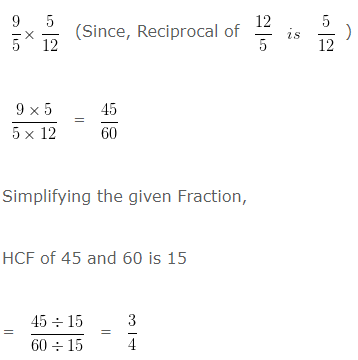# Dividing Fractions

### Dividing Whole numbers by Fractions:

• When a whole number is divided by a fraction, whole number is to be multiplied by the reciprocal of that fraction.
• In generalise form, for any whole number ‘a’ and fraction b/c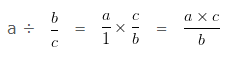• Reciprocal of a fraction, fraction are said to be reciprocal when we simply reverse the fraction i.e numerator becomes denominator and denominator becomes numerator. Two fractions are said to be reciprocal of each other if their product is 1.
• In generalise form, for any fraction a/b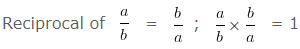Example

Divide 40 by 6/4

Solution

We have, 40 ÷ 6/4

In order to divide a whole number by a Fraction, we need to multiply the whole number with the reciprocal of that Fraction.

We have to multiply Whole number by Reciprocal of that Fraction

40 x 4/6 (Since, Reciprocal of 6/4 is 4/6)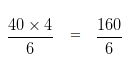Simplifying the given Fraction,

HCF of 160 and 6 is 2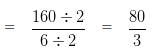### Dividing Fraction by Whole Number:

• When a fraction is divided by a whole number, fraction is to be multiplied by the reciprocal of that whole number.
• In generalise form, for any fraction a/b and whole number ‘c’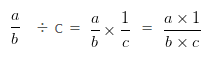Example

Divide 3/7 by 6

Solution

We have, 3/7 ÷ 6

In order to divide a Fraction by a whole number

We have to multiply Fraction with Reciprocal of that whole number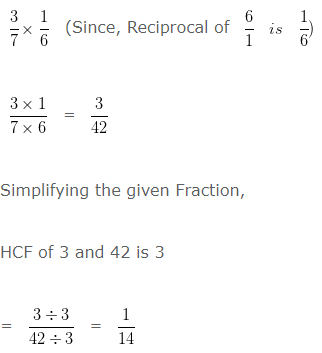### Dividing Fraction by Fractions

• When a fraction is divided by another fraction, the first fraction is multiplied by the reciprocal of second fraction.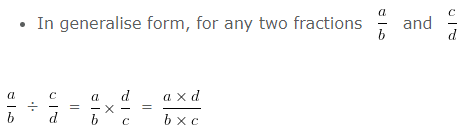Example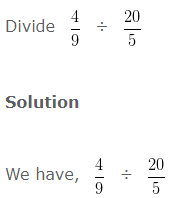In order to divide a Fraction by another Fraction

We have to multiply First Fraction with Reciprocal of the second Fraction### Division of Mixed Fraction by Improper or Proper Fractions

• When a mixed fraction is divide by a proper or improper fraction, convert mixed fraction into improper fraction and then divide them.

Example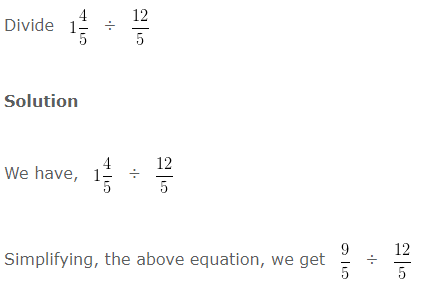In order to divide a Fraction by another Fraction

We have to multiply First Fraction with Reciprocal of the second Fraction